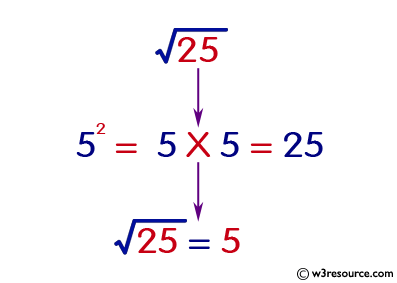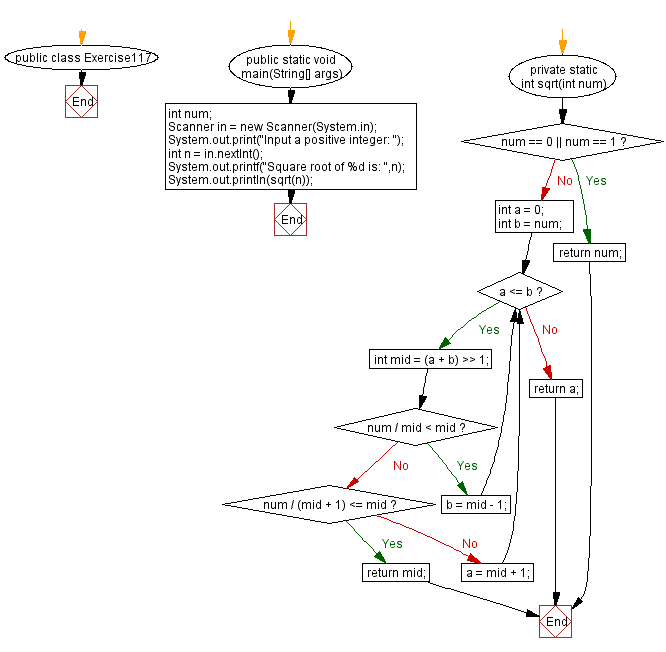﻿ Java exercises: Compute the square root of a given integer - w3resource# Java Exercises: Compute the square root of a given integer

## Java Basic: Exercise-117 with Solution

Write a Java program to compute the square root of a given integer.

Pictorial Presentation:Sample Solution:

Java Code:

``````import java.util.*;
public class Exercise117 {
public static void main(String[] args)
{
int num;
Scanner in = new Scanner(System.in);
System.out.print("Input a positive integer: ");
int n = in.nextInt();
System.out.printf("Square root of %d is: ",n);
System.out.println(sqrt(n));
}

private static int sqrt(int num) {
if (num == 0 || num == 1) {
return num;
}
int a = 0;
int b = num;
while (a <= b) {
int mid = (a + b) >> 1;
if (num / mid < mid) {
b = mid - 1;
} else {
if (num / (mid + 1) <= mid) {
return mid;
}
a = mid + 1;
}
}
return a;
}
}
```
```

Sample Output:

```Input a positive integer: 25
Square root of 25 is: 5
```

Flowchart:Java Code Editor:

What is the difficulty level of this exercise?

Test your Programming skills with w3resource's quiz.

﻿

## Java: Tips of the Day

countOccurrences

Counts the occurrences of a value in an array.

Use Arrays.stream().filter().count() to count total number of values that equals the specified value.

```public static long countOccurrences(int[] numbers, int value) {
return Arrays.stream(numbers)
.filter(number -> number == value)
.count();
}
```

Ref: https://bit.ly/3kCAgLb